•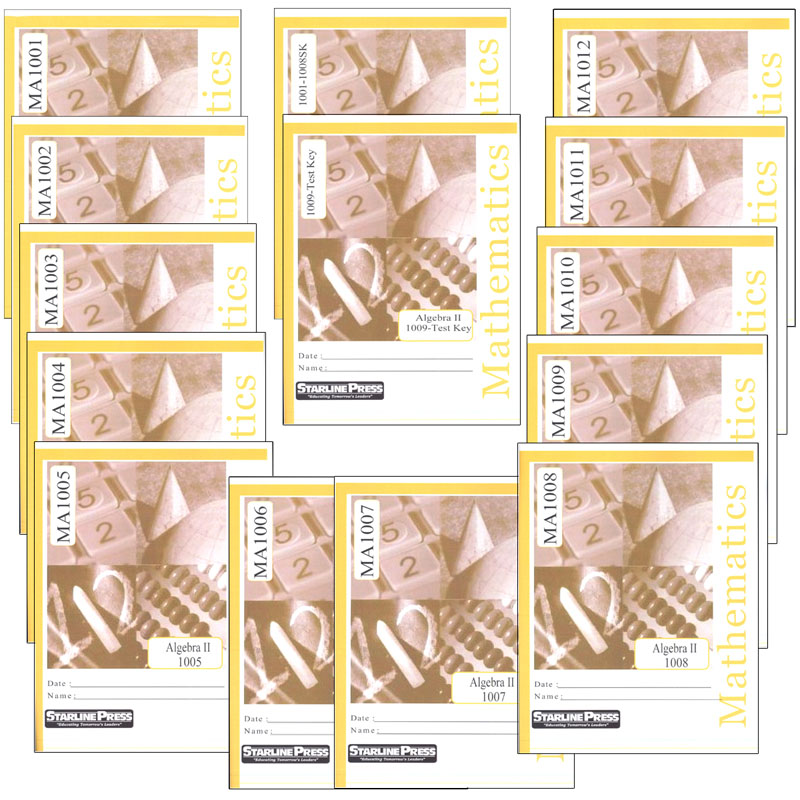•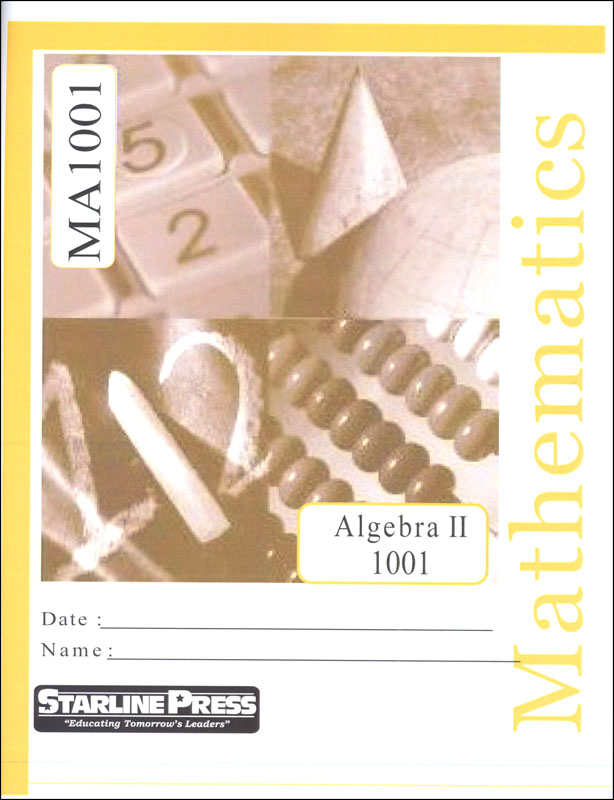•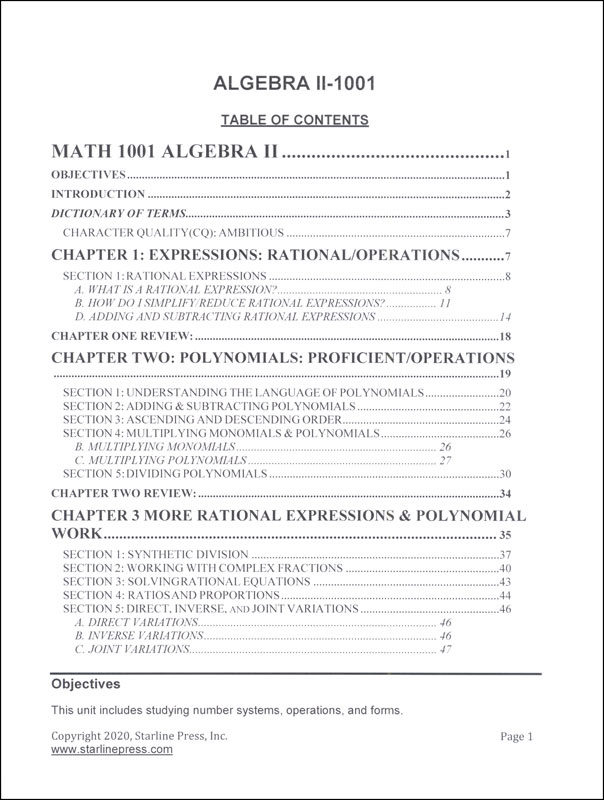•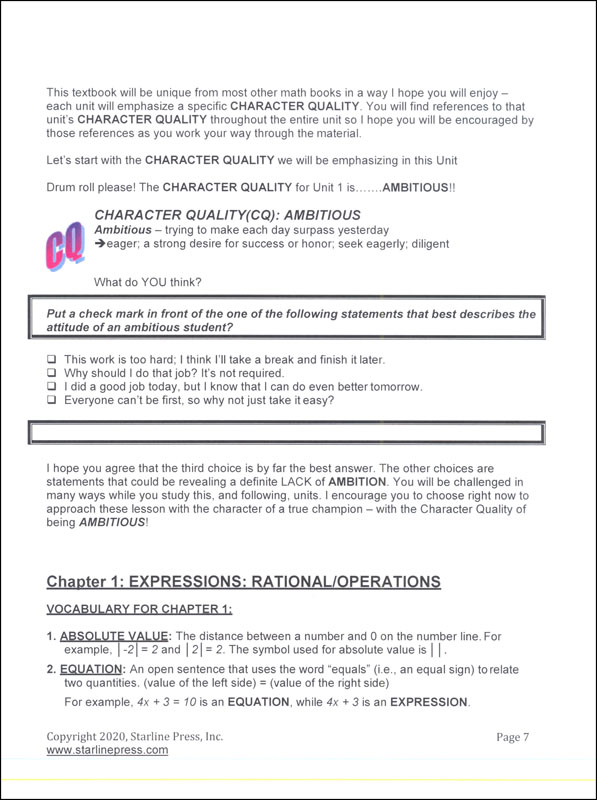•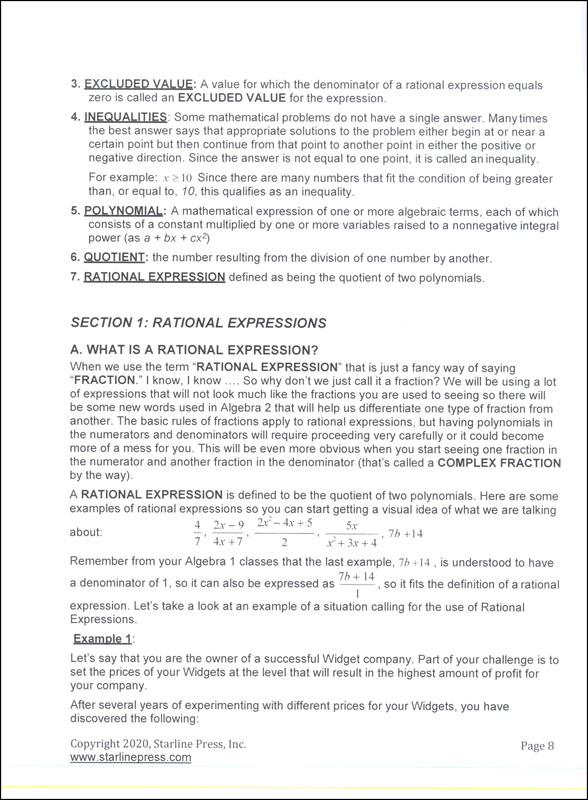•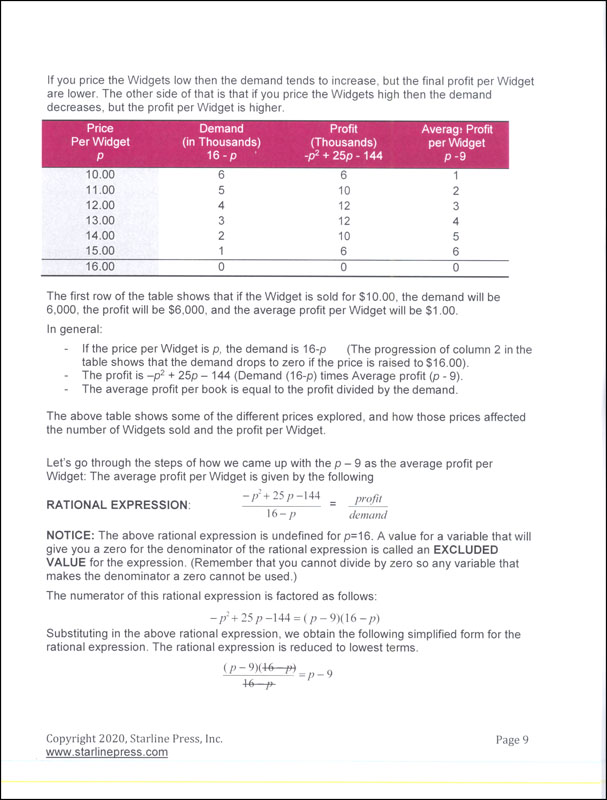•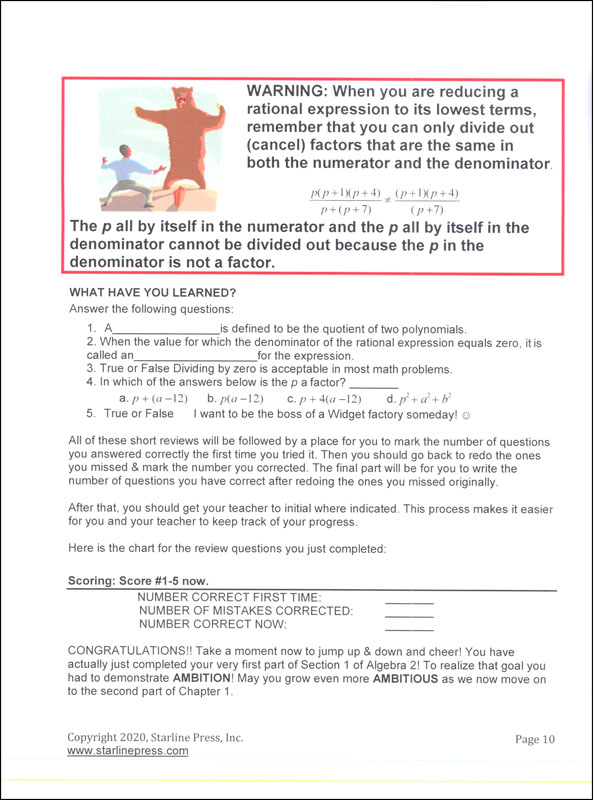# Algebra II Set

# 051442

Our Price: \$199.95
Retail: \$218.71
Save: 8.58% (\$18.76)
In Stock.
Qty:
Qty:

 Item #: 051442 10

#### Product Description:

ALGEBRA II: Students add, subtract, multiply and divide polynomials. They will also work with complex fractions, ratios, and proportions. The basics of quadratic equations, the square root method, and solving absolute value equations are also learned. Students learn about linear inequalities including their addition and multiplication properties. They also study logarithms and antilogarithms and how to work these problems on a calculator. Pascal's Triangle and the Binomial Theorem are studied. Students make and interpret scatter plots, as well as parabola basics and graphing parabolas. See the Scope and Sequence PDF for more details on which topics are covered in each booklet.

#### Publisher Description:

Tenth grade students add, subtract, multiply and divide polynomials. They work with complex fractions, ratios, and proportions. Students study the basics of quadratic equations, the square root method, and solving absolute value equations. Students learn about linear inequality, graphing linear inequalities, applying systems of linear inequalities, and addition and multiplication properties of linear inequalities. Tenth grade students study logarithms and antilogarithms and how to work these problems on a calculator. They study Pascal's Triangle and The Binomial Theorem. Students make and interpret scatter plots, as well as parabola basics and graphing parabolas.

Category Description for Starline Algebra:

Starline Press Algebra is a character-based, individualized and independent learning curriculum. Aligned to pre-Common Core California state standards (please see publisher website for CA state standards correlation), there are 16 student booklets in Algebra 1 and 10 student booklets in Algebra 2 (currently). Each grade level includes vocabulary, fill in the blank questions, practice problems, chapter reviews, and unit tests. Consumable worktexts are colorfully illustrated soft covers, although newer printings are more like booklets and do not have the perfect binding and glossy covers. Available only in Complete Sets which include answer and test keys.

Category Description for Starline Press Math:

Starline Press Math is a character-based, individualized and independent learning curriculum. Aligned to pre-Common Core California state standards (please see publisher website for CA state standards correlation), each grade level provides self- directed instruction with minimal teacher supervision. Grades 3-8 complete twelve units (booklets) per year, with a suggested 3 week completion time frame. Grades 9-10 complete 6 booklets per semester /12 per year. Each grade level includes vocabulary, fill in the blank questions, practice problems, chapter reviews, and unit tests. Consumable worktexts are colorfully illustrated soft covers, although newer printings are more like booklets and do not have the perfect binding and glossy covers. Available only in complete sets which include answer and test keys. ~ Deanne

Category Description for COMPREHENSIVE PROGRAMS - ALL GRADES:

Primary Subject
Mathematics
10
Format
Softcover Book Set
Brand Name
Starline Press
Weight
4.55 (lbs.)
Dimensions
11.0" x 8.75" x 2.0"